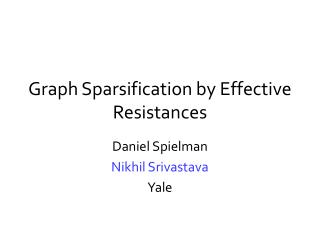DownloadDownload PresentationGraph Sparsification by Effective Resistances

# Graph Sparsification by Effective Resistances

Télécharger la présentation## Graph Sparsification by Effective Resistances

- - - - - - - - - - - - - - - - - - - - - - - - - - - E N D - - - - - - - - - - - - - - - - - - - - - - - - - - -
##### Presentation Transcript

1. Graph Sparsification by Effective Resistances Daniel Spielman Nikhil Srivastava Yale

2. Sparsification Approximate any graph G by a sparse graph H. • Nontrivial statement about G • H is faster to compute with than G G H

3. Cut Sparsifiers [Benczur-Karger’96] H approximates G if for every cut S½V sum of weights of edges leaving S is preserved Can find H with O(nlogn/2) edges in time S S

4. The Laplacian (quick review) Quadratic form Positive semidefinite Ker(LG)=span(1) if G is connected

5. Cuts and the Quadratic Form For characteristic vector So BK says:

6. A Stronger Notion For characteristic vector So BK says:

7. Why?

8. 1. All eigenvalues are preserved By Courant-Fischer, G and H have similar eigenvalues. For spectral purposes, G and H are equivalent.

9. 1. All eigenvalues are preserved By Courant-Fischer, G and H have similar eigenvalues. For spectral purposes, G and H are equivalent. cf. matrix sparsifiers [AM01,FKV04,AHK05]

10. 2. Linear System Solvers Conj. Gradient solves in ignore (time to mult. by A)

11. 2. Preconditioning Find easy that approximates . Solve instead. Time to solve (mult.by )

12. 2. Preconditioning Use B=LH ? Find easy that approximates . Solve instead. ? Time to solve (mult.by )

13. 2. Preconditioning Spielman-Teng [STOC ’04] Nearly linear time. Find easy that approximates . Solve instead. Time to solve (mult.by )

14. Examples

15. Example: Sparsify Complete Graph by Ramanujan Expander G is complete on n vertices. H is d-regular Ramanujan graph.

16. Example: Sparsify Complete Graph by Ramanujan Expander G is complete on n vertices. H is d-regular Ramanujan graph. So, is a good sparsifier for G. Each edge has weight (n/d)

17. Example: Dumbell Kn Kn 1 d-regular Ramanujan, times n/d d-regular Ramanujan, times n/d 1

18. G2 Kn Kn G1 G3 1 F2 d-regular Ramanujan, times n/d d-regular Ramanujan, times n/d 1 F1 F3 Example: Dumbell

19. Example: Dumbell. Must include cut edge Kn Kn e Only this edge contributes to If

20. Results

21. Main Theorem Every G=(V,E,c) contains H=(V,F,d) with O(nlogn/2) edges such that:

22. Main Theorem Every G=(V,E,c) contains H=(V,F,d) with O(nlogn/2) edges such that: Can find H in time by random sampling.

23. Main Theorem Every G=(V,E,c) contains H=(V,F,d) with O(nlogn/2) edges such that: Can find H in time by random sampling. Improves [BK’96] Improves O(nlogc n) sparsifiers [ST’04]

24. How? Electrical Flows.

25. Effective Resistance Identify each edge of G with a unit resistor is resistance between endpoints of e 1 v u 1 1 a

26. Effective Resistance Identify each edge of G with a unit resistor is resistance between endpoints of e 1 v u Resistance of path is 2 1 1 a

27. Effective Resistance Identify each edge of G with a unit resistor is resistance between endpoints of e 1 v u Resistance from u to v is Resistance of path is 2 1 1 a

28. Effective Resistance Identify each edge of G with a unit resistor is resistance between endpoints of e -1 +1 v u 1/3 -1/3 a 0

29. Effective Resistance Identify each edge of G with a unit resistor is resistance between endpoints of e V +1 -1 = potential difference between endpoints when flow one unit from one endpoint to other

30. Effective Resistance V +1 -1 [Chandra et al. STOC ’89]

31. The Algorithm Sample edges of G with probability If chosen, include in H with weight Take q=O(nlogn/2) samples with replacement Divide all weights by q.

32. An algebraic expression for Orient G arbitrarily.

33. An algebraic expression for Orient G arbitrarily. Signed incidence matrix Bm£ n :

34. An algebraic expression for Orient G arbitrarily. Signed incidence matrix Bm£ n : Write Laplacian as

35. V +1 -1 An algebraic expression for

36. An algebraic expression for Then Reduce thm. to statement about 

37. Goal Want

38. Sampling in 

39. Reduction to  Lemma.

40. New Goal Lemma.

41. The Algorithm Sample edges of G with probability If chosen, include in H with weight Take q=O(nlogn/2) samples with replacement Divide all weights by q.

42. The Algorithm Sample columns of with probability If chosen, include in with weight Take q=O(nlogn/2) samples with replacement Divide all weights by q.

43. The Algorithm Sample columns of with probability If chosen, include in with weight Take q=O(nlogn/2) samples with replacement Divide all weights by q.

44. The Algorithm Sample columns of with probability If chosen, include in with weight Take q=O(nlogn/2) samples with replacement Divide all weights by q.

45. The Algorithm Sample columns of with probability If chosen, include in with weight Take q=O(nlogn/2) samples with replacement Divide all weights by q. cf. low-rank approx. [FKV04,RV07]

46. A Concentration Result

47. A Concentration Result So with prob. ½:

48. A Concentration Result So with prob. ½: